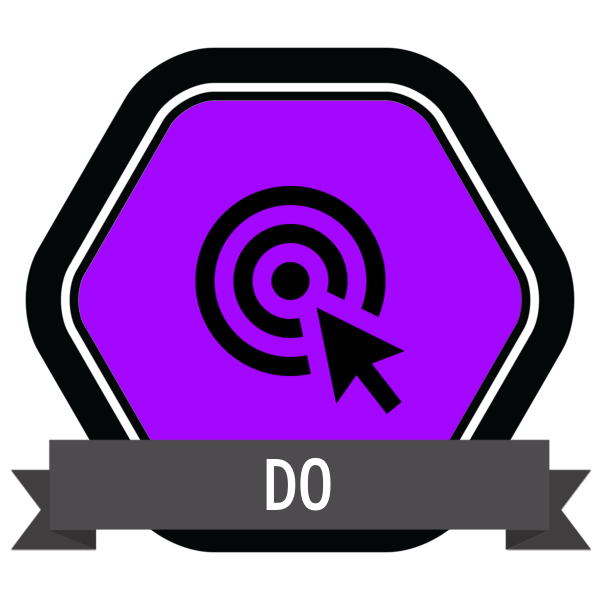# The Metron

Students will demonstrate the ability to measure matter involving one variable (i.e. g, kg, m, s, hr, ml, L, cm, km, celsius) of matter in at least two states (solid, liquid, gas).

Skills range:

Accurately measure commonly used lab substances.

Demonstrate accurate measurements across at least two of three states:

Liquid, (Gas), Solids, Energy

In doing so, you should show an understanding of at least two of the following variables:

Mass     Volume     Length     Time      Temperature

Possible equipment:

• Measuring cylinder
• Tablespoon
• Scales
• Stopwatch
• Thermometer
• Ruler
• Gas syringe
• Ammeter
• Voltmeter

Criteria:

1. Select the correct equipment
2. Accurately read from a scale
3. Select correct units for measurement

Context:

This skill is practical-based and therefore needs to be observed by a teacher/mentor/technician by using appropriate laboratory equipment and showing appropriate calculations.

Safety:

Conscious of actions in the lab environment

Knowledge of hazards

Exemplar:Students will demonstrate the ability to measure matter involving one variable (i.e. g, kg, m, s, hr, ml, L, cm, km, celsius) of matter in at least two states (solid, liquid, gas). Skills range: Accurately measure commonly used lab substances. Demonstrate accurate measurements across at least two of three states:  Liquid, (Gas), Solids, Energy In doing so, you […]
FIRST PUBLISHED: July 31, 2020
COMPETENCIES:
SUBJECT: Mathematics | Science